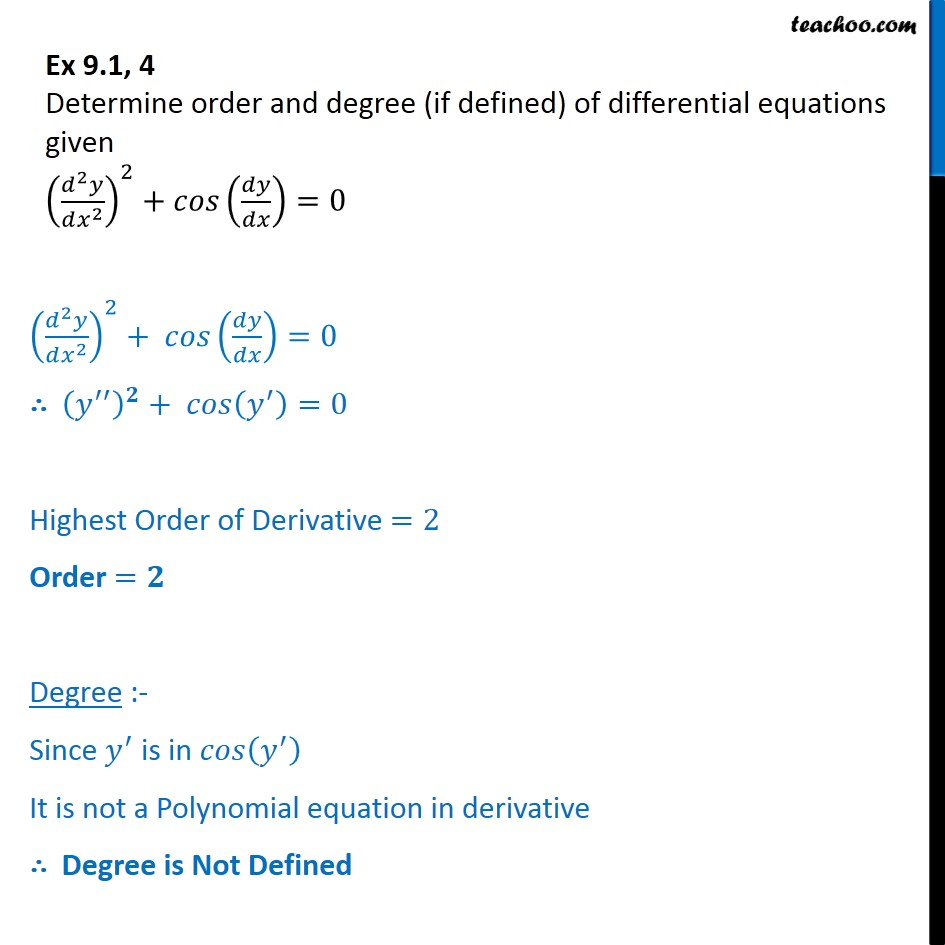Ex 9.1

Chapter 9 Class 12 Differential Equations
Serial order wiseLearn in your speed, with individual attention - Teachoo Maths 1-on-1 Class

### Transcript

Ex 9.1, 4 Determine order and degree (if defined) of differential equations given (d2y/dx2)2 + cos (dy/dx) = 0 (d2y/dx2)2 + cos (dy/dx) = 0 ∴ (y'')2 + cos (y') = 0 Highest Order of Derivative =2 Order =𝟐 Degree :- Since y' is in 𝑐𝑜𝑠 (y') It is not a Polynomial equation in derivative ∴ Degree is Not Defined